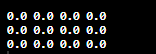# 1) Write Java code to declare a two dimensional array of 3 columns and 4 rows. The array will hold doubles.double [][] list = new double ;What is the array initialized to by default? 2) Write Java code to find the lowest value in a 2 dim array.

Question
8 views

1) Write Java code to declare a two dimensional array of 3 columns and 4 rows. The array will hold doubles.

double [][] list = new double ;

What is the array initialized to by default?

2) Write Java code to find the lowest value in a 2 dim array.

check_circle

Step 1

Note: We are authorized to answer only one question at a time. Since you have asked more than one question at the time, we are answering the first question only. Please, re-post your question separately for the remaining question.

Program plan:

• Declare the two-dimension array in java program.
• Print the two-dimension array without assigning any value in it.
• The value printed after this is the array initialed by default.
Step 2

Program:

//class defaultinItialized

public class defaultinItialized  {

//main method

public static void main(String args[]) {

//declare a two dimensional array of 3 columns and 4 rows

double [][] list = new double ;

//outer for loop for rows

for(int i=0;i<list.length;i++)

{

//inner for loop for columns

for(int j=0;j<list[i].length;j++)

{

//printing array initialized to by default

System.out.print(list[i][j]+" ");

}

//printing new line

System.out.println();

}

}

}

Step 3

Sample Output:...

### Want to see the full answer?

See Solution

#### Want to see this answer and more?

Solutions are written by subject experts who are available 24/7. Questions are typically answered within 1 hour.*

See Solution
*Response times may vary by subject and question.
Tagged in